# Non-parametric survival analysis

May 22, 2018 - 12 min read

In biomedical research, especially in the fields of epidemiology or oncology, one of the most common outcome under assessment is the time to an event of interest (also called “failure”), namely survival time. The considered event is often death, but could be anything else such as cancer relapse or progression instead. The vast majority of survival analyses have extensively been using Kaplan-Meier (KM) estimates, log-rank tests and Cox proportional hazards (CoxPH) models, all of which we will describe shortly. This post is an attempt to review the most classical approach to survival analyses, in prevision for a following post discussing how everything fares in the high dimensional setting and solutions to potential problems that might arise.

## Survival setting and notations

One difficulty arising when analysing time-to-event data is that not all subjects experimented the said event: survival times will be unknown for a subset of the study group. This phenomenon is called censoring, of which there are three types (left, right and interval censoring). The Cox model only considers right-censored data: sometimes, a patient will drop out of the study (i.e., voluntarily or because he was still alive at the end of the study) and we only analyse the participants that haven’t had the event at the start of the study.

Let $T$ be a continous random variable representing survival time, with probability density function $f(t)$ and cumulative distribution function $F(T)$. The survival function $S(t)$ is the probability of a patient surviving from the time origin (e.g. diagnosis of cancer) to a specified future time $t$, ie.,

$S(t) = P(T\geq t) = 1-F(T) = \int_t^\infty f(x)dx$

Another important function is the hazard function, denoted $\lambda(t)$ (or often also $h(t)$), which is the instant probability that the event occurs right at time $t$:

$\lambda(t) = \lim_{dt\rightarrow0} \frac{P(t \le T < t + dt | T \geq t ) }{dt}$

To be a little bit more precise, the numerator corresponds to the conditional probability that the event will occur in the small interval $[t, t+dt)$ given that it has not occurred before. The whole expression is then equivalent to the rate of event occurrence per unit of time.

From the previous definitions, it follows that

\begin{aligned} \lambda(t) &= \lim_{dt\rightarrow0} \frac{P(t \le T < t + dt, T \geq t ) / P(T \geq t)}{dt} \\ &= \lim_{dt\rightarrow0} \frac{f(t)dt / S(t)}{dt} \\ &= \frac{f(t)}{S(t)} \end{aligned}

By noticing that $-f(t)$ is the derivative of $S(t)$, we can rewrite our last result as

$\lambda(t) = - \frac{d}{dt}\log S(t)$

Finally, by integrating from $0$ to $t$ and introducing the boundary condition $S(0)=1$ (by definition, no event occurred yet at time $t=0$), we get the following expression:

\begin{aligned} S(t) &= \exp \left( - \int_0^t \lambda(x)dx \right) \\ &= \exp \left( - \Lambda(t) \right) \end{aligned}

where $\Lambda(t)$ is called the cumulative hazard and is interpretable as the sum of the risks faced going from $t=0$ to $t=t$.

In summary, we can say that while the hazard relates to the incident (current) event rate and survival reflects the cumulative non-occurrence, both provide equivalent characterizations of the distribution $T$. Given the survival function, we can always differentiate to obtain the density and then calculate the hazard. Given the hazard, we can always integrate to obtain the cumulative hazard and then exponentiate to obtain the survival function.

## Kaplan-Meier estimation

However, estimation of the survival function is not trivial. If the data were not censored, the obvious estimate would be the empirical survival function derived from our data

$\hat{S}(t) = \frac1n \sum_{i=1}^n \mathbb{I}_{t_i > t}$

where $\mathbb{I}_{t_i > t}$ is the indicator function that takes the value $1$ if the associated condition is true and $0$ otherwise. The estimator is then simply the proportion of participants alive (ie. that did not experiment the event) at time $t$. But due to censoring, we do not have the time of event $t_i$ for all patients and therefore must find another way to estimate the survival function. Kaplan and Meier (1958) came up with a way to do that elegantly.

Let $t_{(1)} < t_{(2)} < ... < t_{(m)}$ denote the distinct ordered times of events, and let $n\_i$ be the number alive just before $t_{(i)}$; $n_i$ is considered the number exposed to risk at time $t\_i$. The Kaplan-Meier estimator of the survival function is

$\hat{S}(t) = \prod_{i:t_{(i)} < t} \left( 1 - \frac{d_i}{n_i} \right)$

which is really just a product of the observed probability to survive at time $t_{(1)}$ times the probability to survive at time $t_{(2)}$ conditioned on having survived at time $t_{(1)}$, and so on. The value of $\hat{S}(t)$ being constant between times of events, the estimated probability is a step function that changes value only at the time of each event. Interestingly enough, this estimator actually corresponds to the non-parametric maximum likelihood estimator (MLE) of the survival function. Furthermore, if there is no censoring, the KM estimate coincides with the empirical survival function.

The KM survival curve, a plot of the KM survival probability against time, provides a useful summary of the data that can be used to estimate measures such as median survival time. As an example, we’ll be using a dataset of 42 leukemia patients in a clinical trial to compare treatment with placebo (from Freireich et al., 1963).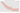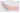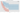It is good practice to also report the risk count table under the curves, which indicates at each time the number of participants at risk of having the event. It was omitted here for the sake of tidyness.

The Kaplan-Meier curves are a really useful way to represent survival analyses, as they speak for themselves and are relatively easy to interprete. This quality is one of the main reasons that made them so widespread in the medical research area. They are often accompanied by a p-value that is obtained by a formal test we’ll be discussing now.

## Log-rank test

Survival in two or more groups can be compared by formal statistical tests, among which the log-rank test (Peto et al., 1977) is the most common by far—so common in fact, that you’re expected to justify it in your paper if you’re using another one. It is a non-parametric test used to test the null hypothesis that there is no difference between the populations in the probability of an event at any time point. At each time of events and for each group, we calculate the number of events one would expect since the previous event if there were no differences between the groups, and compare them to the observed number of events with the following statistic

$\chi^2 = \sum_{g=1}^G \frac{(O_g - E_g)^2}{E_g}$

where $G$ is the number of groups that are being compared, $O_g$ the sum of all observed events and $E_g$ the sum of all expected events given by

$E_g = \sum_{i=1}^{m} \frac{O_{t_{(i)}}}{N_{t_{(i)}}}N_{g,t_{(i)}}$

where each $t_{(i)}$ is a time of events and $N_{t_{(i)}}$ the number of participants at risk of having the event at that time.

This value is then compared to a $\chi^2$ distribution with $G-1$ degrees of freedom to compute a p-value to be interpreted—we say that significant difference has been found between the hazard functions estimates if $p < \text{threshold}$ (usually $0.05$). In the setting where we compare only two groups, the null hypothesis of the test is equivalent to saying that the ratio of the hazard rates is equal to one; this hazard ratio (HR) is a measure of the relative survival experience in the two groups

$H_0: \text{HR}\approx\frac{O_1/E_1}{O_2/E_2}=1$

where $O_g/E_g$ is the estimated relative excess hazard in group $g$. This will serve as a very brief introduction to the important notion of hazard ratio; in practice, it is better to estimate it using a regression modelling technique such as the Cox proportional hazards model we will study shortly.

VariableLog-rank $\chi^2$ statisticp-value
Gender$0.5569$$0.4555$
Treatment$16.7929$$<10^{-4}$

Above are the log-rank tests for the plots we saw in the previous section. Our visual intuitions have indeed been comforted by the tests results: we were not able to detect a difference in survival between genders, but there is a significant one between the placebo and the treatment groups. Looking back at the plots, we can say that the highlighted difference is in favor of the treatment group. Because the logrank test is purely a test of significance it cannot provide an estimate of the size of the difference between the groups or a confidence interval.

## Interlude: assumptions

Even if the Kaplan-Meier estimate and the log-rank test are considered non-parametric, they still rely on non-trivial assumptions. Luckily, they are the same for both:

1. At any time, patients who are censored should have the same survival prospects as those who continue to be followed—ie. censoring should not be related to prognosis.

2. The survival probabilities should be the same for subjects recruited early and late in the study.

3. We assume that the event happens at the time specified. This is often not the case for events happening between two examinations for example.

These three conditions are seldom completely fulfilled; this rarely forbids the use of the above mentioned methods, but this can lead to some bias in the estimations and should be reported in the papers.

## Cox proportional hazards model

Initially, this post was only supposed to be about about the CoxPH model. But as I started writing, it seemed weird not to have an introduction to survival analyses in general, so I decided to write the previous parts as an introduction to what I think is the core statistical model of a huge part of modern medical research—the Cox proportional hazard model (Sir David Cox, 1972).

Suppose that instead of studying the impact of just one variable on survival we now have a vector $\boldsymbol{x}$ of $p$ covariates. The hazard at time $t$ for an individual is assumed here to be

$\lambda(t|\boldsymbol{x}) = \lambda_0(t) \exp(\boldsymbol{x}\boldsymbol{\beta} )$

where $\boldsymbol{\beta}$ is a $p \times 1$ vector of unknown parameters and $\lambda_0(t)$ is an unknown baseline hazard function for all individuals corresponding to the case were all covariates $\boldsymbol{x}=0$.

As before, $t_{(1)} < t_{(2)} < ... < t_{(m)}$ denote the distinct ordered times of events. We’ll first consider the simple case where there are no ties—ie. only one subject had the event at $t_{(i)}$ (if you wish to know how the Cox model handles ties, please see here)—and $R_i$ denotes the the set of indices of participants at risk at time $t_{(i)}$ (that are alive just before $t_{(i)}$). For the subject $j_{(i)}$ that had the event at time $t_{(i)}$, its probability of failing at this specific time conditionally on the risk set is then as follows

$\frac{\lambda_0(t_{(i)}) \exp(\boldsymbol{x}_{j_{(i)}}\boldsymbol{\beta} )}{\sum_{j \in R_i}\lambda_0(t_{(i)}) \exp(\boldsymbol{x}_j\boldsymbol{\beta} )}$

That’s where the magic happens: the annoying baseline hazard term cancels out and we’re left with only

$\frac{ \exp(\boldsymbol{x}_{j_{(i)}}\boldsymbol{\beta} )}{\sum_{j \in R_i}\exp(\boldsymbol{x}_j\boldsymbol{\beta} )}$

As only failures contribute to the hazard function, the likelihood function, which is called a “partial” likelihood because we won’t bother estimating the baseline hazard function, is then just

$L=\prod_{i=1}^m\frac{ \exp(\boldsymbol{x}_{j_{(i)}}\boldsymbol{\beta} )}{\sum_{j \in R_i}\exp(\boldsymbol{x}_j\boldsymbol{\beta} )}$

And its log-likelihood has the form

$\log{L}=\sum_{i=1}^m \left(\boldsymbol{\beta}\boldsymbol{x}_{j_{(i)}}\boldsymbol{\beta} - \log \sum_{j \in R_i}\exp(\boldsymbol{x}_j\boldsymbol{\beta} ) \right)$

Efron (1977) showed that even though the partial likelihood is not a true likelihood, it loses little to no information for a wide variety of hazard functions and that it can be treated as a likelihood for asymptotic inference. This lets us derive maximum likelihood estimates and asymptotic confidence intervals the usual way.

We can already summarize three very attractive features of Cox’s approach:

1. The “nuisance” function $\lambda_0(t_{(i)})$ is completely removed from the inference process on $\boldsymbol{\beta}$.

2. We can model the effect of multiple covariates at the same time in the $\exp(\boldsymbol{x}\boldsymbol{\beta} )$ term.

3. Data censoring does not affect the likelihood function, as it only depends on participants that experimented the event.

The only thing left now is to estimate the $\beta$ coefficients for all covariates via maximum likelihood estimation (MLE), which can be done effectively by numerous algorithms such as the Expectation Maximization (EM) algorithm (such as here and here).

You will then get the following output, even though it might slightly differ depending on the statistical software and/or librairies you’re using:

Cox-PH model summary:
n = 42, number of events = 30
Variablecoefexp(coef)se(coef)$z$$p$$\downarrow$ 0.95$\uparrow$ 0.95
Gender0.31471.36980.45450.69230.4887-0.57611.2055
Treatment1.50364.49780.46153.25800.00110.59902.4081
logWBC1.68195.37600.33664.99710.00001.02232.3416
Concordance = 0.851

The coefficients in a Cox regression relate to hazard; a positive coefficient indicates a worse prognosis and a negative coefficient indicates a protective effect of the variable with which it is associated.

The hazards ratio associated with a predictor variable is given by the exponent of its coefficient, with $\text{HR}<1$ indicating protective effect, $\text{HR}=1$ no influence and $\text{HR}>1$ worse prognosis. It can be seen as a relative event rate and its interpretation depends on the measurement scale of the covariate in question; an increase in $x$ units leads to an increase in hazard rate of $x \times\text{HR}$ if all other covariates remain the same. If we take the Treatment variable, as it is binary ($0$ for treatment, $1$ for placebo), we can say that receiving a placebo (ie. an increase of one unit) leads to an increase in $4.5$ of the hazard rate (ie. at all times, an individual from the placebo group has $4.5\times$ more chance to have the event than an individual from the treatment group that has the same gender and logWBC). If we take the logWBC variable (logarithm of the white blood cells count), we see that an increase in one unit leads to an increase in $5.4$ in the hazard rate. More broadly, if we consider the two following individuals

IDGenderTreatmentlogWBC
1Femaleplacebo1
2Maletreatment3

then their hazard rate can be expressed as

\begin{aligned} \lambda(t|\boldsymbol{x}_1) &= \lambda_0(t) \exp(\boldsymbol{x}_1\boldsymbol{\beta} ) \\ &= \lambda_0(t) \exp\left(x_{fem} \times \beta_{Gen} + x_{pla} \times \beta_{Treat} + x_1 \times \beta_{WBC}\right) \\ \end{aligned}
\begin{aligned} \lambda(t|\boldsymbol{x}_2) &= \lambda_0(t) \exp(\boldsymbol{x}_2\boldsymbol{\beta} ) \\ &= \lambda_0(t) \exp\left(x_{mal} \times \beta_{Gen} + x_{treat} \times \beta_{Treat} + x_3 \times \beta_{WBC}\right) \\ \end{aligned}

and their hazard ratio is then

\begin{aligned} \text{HR}_{\boldsymbol{x}_1 / \boldsymbol{x}_2} &= \frac{\exp\left(x_{fem} \times \beta_{Gen} + x_{pla} \times \beta_{Treat} + x_1 \times \beta_{WBC}\right)}{\exp\left(x_{mal} \times \beta_{Gen} + x_{treat} \times \beta_{Treat} + x_3 \times \beta_{WBC}\right)} \\ &= \frac{\exp\left(0 \times 0.3147 + 1 \times 1.5036 + 1 \times 1.6819\right)}{\exp\left(1 \times 0.3147 + 0 \times 1.5036 + 3 \times 1.6819\right)} = 0.1136 \end{aligned}

thus individual $2$ has $1/0.1136=8.8$ times more chance of having the event (ie. leukemia relapse) than individual $1$.

Back to our Cox-model summary, the next value is the standard error of our coefficient estimate. Without going into too much details, it is calculated from the Fisher information matrix obtained by taking the negative of the second derivative of our log-likelihood with respect to the coefficient of our covariate of interest. The inverse of this matrix is in fact the covariance matrix of our coefficient; summing over the diagonal and taking the square root gives us the standard error.

The p-value of our coefficient is commonly given by a Wald test, which $\boldsymbol{z}$ statistic is calculated by $z=\beta / se(\beta)$ and then compared to a normal distribution. The lower and upper bounds of the $95\%$ confidence interval is just $\beta \pm 1.96 \times se(\beta)$. If the p-value is lower than a threshold (usually $0.05$), then we can say that our coefficient is significantly different than $0$ (equivalent to saying that our HR is significantly different than $1$) and that our corresponding covariate is associated with the event rate. If not, then we cannot say for sure that the value we estimated is actually reflecting the real value or not given our sample; in our example, the p-value for the variable Gender is non significant, which means we cannot say much about its relation to the event rate. It doesn’t mean that it’s not related—maybe we just lack power and it would be significant with more participants in our study.

## Interlude: assumptions

I lied a little with the title of this blog post: the Cox proportional hazards model is not totally non-parametric. Our model contains two unknown parameters, $\lambda_0 (t)$ and $\boldsymbol{\beta}$, the former being an infinite-dimensional component, but the latter being finite-dimensional. We call such a model where both coexist semiparametric. Therefore, on top of the ones already listed above in the previous interlude, it relies on another parametric assumption, namely the proportional hazards assumption.

I’ve already mentioned it several times; when I gave an interpretation for hazard ratios, I wrote that the difference in hazard rates was effective at all times. This is what the proportionality assumption is. Putting it another way, the relative risk of two individuals with different covariate values is independent of time. For example, if the variable considered is Age, it would mean that the hazard ratio for the considered event between a 25y.o and a 28y.o participants is the same as the one between a 95y.o and a 98y.o participants (increase of 3 units of age in both cases).

There are several ways to check that this assumption holds—Schoenfeld, Martingale, deviance and Cox-Snell residuals, etc.—but I personally find the plotting of the log cumulative hazards more attractive visually and more easily interpretable at one glance. These are commonly nicknamed “log-log” plots because we plot the log cumulative hazard against the log of time. Recall from above at each time $t$:

$\log\hat{\Lambda}(t) = \log(-\log(\hat{S}(t))) = \log(-\log(\hat{S}_0(t)))+\hat{\beta} x$

Thus, if the proportional hazards hold, the log cumulative hazards for each group should differ by the same constant—ie. the curves should be parralel.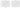So now, what to do when there are covariates that do not satisfy the PH assumption? We will just shortly mention the two most common options out there: either stratifying the model according to the concerned variables (if there’s just a few), or introducing time-dependent effects. The former method assumes PH in each strata, while the latter lets the impact of covariates vary over time. Both are easily implemented in common statistical softwares.

Two other assumptions for the Cox model are made explicit by the formulation of the hazard function: it assumes linear combination of the covariates, as well as the fact that the link function is exponential (cf. generalized linear models).

## Evaluation of the model

Now that we have our model, how do we assess if our model is actually good or not? If it fits the data well enough?

The most widespread statistic to measure goodness-of-fit is Harrell’s C-index, or concordance index or C-statistic. Remember the little “concordance” below our model summary table? One can calculate the C-index by taking all possible pairs of subjects consisting of one subject who experienced the event of interest and one subject who did not experience it. The C-index is the proportion of such pairs in which the subject who experienced the event had a higher predicted probability of experiencing the event than the subject who did not experience it. It is in fact identical to the Receiver Operating Characteristic (ROC) area under curve (AUC) for the binary outcome, and their scale of interpretation is the same:

• 0.5 indicates a model that does no better than chance
• 0.7 indicates a good model
• 0.8 indicates a strong model
• 1.0 indicates a perfect model

Many papers only report the C-statistic as a goodness-of-fit index of their model, but as stated by Steryerberg et al., it is not enough to capture all aspects of it. The C-statistic is a good measure of the discrimination of a model—ie. the ability to discriminate between those with and those without the outcome—but other metrics should be reported alongside it such as calibration plots or the Hosmer-Lemeshow test that measure calibration of a model (agreement between observed outcomes and predictions). When possible, overall performance should also be evaluated on external data that was not used to fit the model.

## Interpretation pitfalls

Many biases can be introduced from the design of the study, and should always be taken into account when interpreting the results of survival analyses (and of any other kind of analysis in general). In clinical trials for example, proper randomization of treatment assignment is not always possible, and can lead to serious selection biases, ie. the samples obtained are not representative of the population intended to be analyzed. Among those, one of the most prominent is the survival bias or survivorship bias, where the sample is only represented by participants that survived up to some point. An excellent (and cynical) example of its consequences can be found in this correspondance to the authors of a biased paper published in one of the most influential oncology journal; in a secondary analysis, they compared three treatment durations and concluded that longer treatment increased overall, disease-free and progression-free survival. However, patients who received treatment for more than 5 years (ie, group 3) first had to live for 5 years—ie. group 3 was composed of subjects that were maybe just inherently more resistant than the two other groups. The following plots were simulated by the authors of the correspondance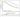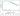There are many other types of biases one should be aware of when analysing results, especially in secondary analyses as study designs are usually conceived with only primary outcomes in mind and are often ill-suited for others, but they relate to statistical biases in general and would take a whole post to write about.

## Conclusion

This concludes my review of classical methods commonly found in survival analyses. I’ll admit it was a bit lengthier than I expected; I initially planned to write about recent improvements (or at least tentatives) in machine learning and deep learning for survival analyses in the same post, but I guess I’ll leave it for later. I tried to be as exhaustive as possible while keeping it simple and clear.

All code from this post can be found in a notebook on my github here.